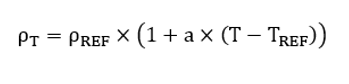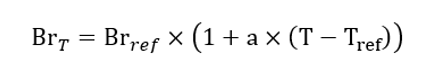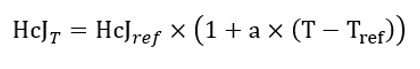# General relations with temperature

## 1. Resistivity

Note: Only isotropic materials are considered.
Note: Resistivity ρ (rho) is a linear function of temperature.

The corresponding mathematical formula is:ρ T Resistivity to be defined at a temperature T. Linear function of the temperature for an isotropic or anisotropic material. T REF Reference temperature. T T is the temperature for which the resistivity must be computed. ρ REF Resistivity of the material at T REF . a Temperature coefficient at T REF .

## 2. Thermal conductivity for all materials except gas and liquid

The thermal conductivity is defined at a reference temperature and is considered as constant for all thermal computations.

The reference temperature is then only a memo, to keep in mind the temperature corresponding to the indicated thermal conductivity.

 Symbol Definition Unit T ref Reference temperature (Tref) °C K ref Isotropic thermal conductivity at Tref W/K/m) W/K/m

## 3. Specific heat variation versus temperature – For all material except gas and liquid

The specific heat is defined at a reference temperature and is considered as constant for all thermal computations.

The reference temperature is then only a memo, to keep in mind the temperature corresponding to the indicated specific heat.

 Symbol Definition Unit T ref Reference temperature (Tref) °C C ref Specific heat at Tref (J/K/Kg) J/K/Kg

## 4. Remanent induction of magnets

Note: Only isotropic magnet is considered.
Note: Remanent induction (Br) is a linear function of the temperature.

The corresponding mathematical formula is:Br T Remanent induction to be defined at a temperature T. Linear function of the temperature for an isotropic or anisotropic material. T ref Reference temperature. T T is the temperature for which the remanent induction must be computed. Br ref Remanent induction of the magnet at T REF . a Reverse temperature coefficient for Br at T REF .

## 5. Intrinsic Coercivity

Note: Only isotropic magnet is considered.
Note: Intrinsic Coercivity (HcJ) is a linear function of the temperature.

The corresponding mathematical formula is:HcJ T Intrinsic Coercivity to be defined at a temperature T. Linear function of the temperature for an isotropic or anisotropic material. T ref Reference temperature. T T is the magnet temperature for which the Intrinsic Coercivity must be computed. HcJ ref Intrinsic Coercivity of the magnet at T REF . a Reverse temperature coefficient for Hcj at T REF .